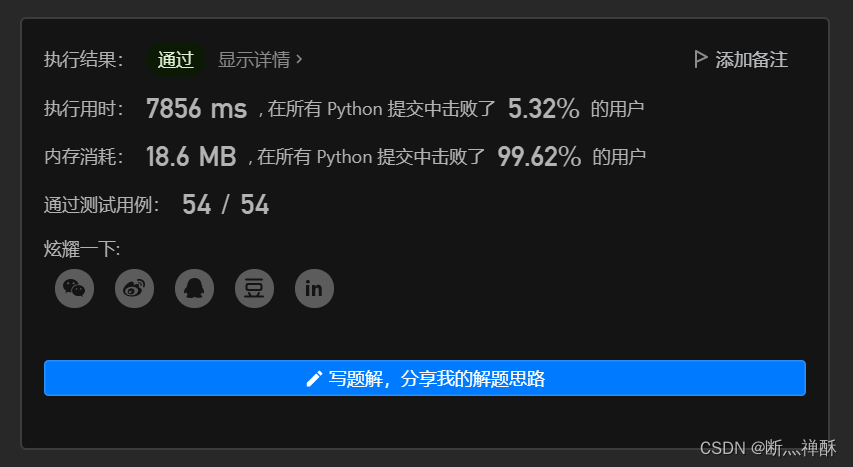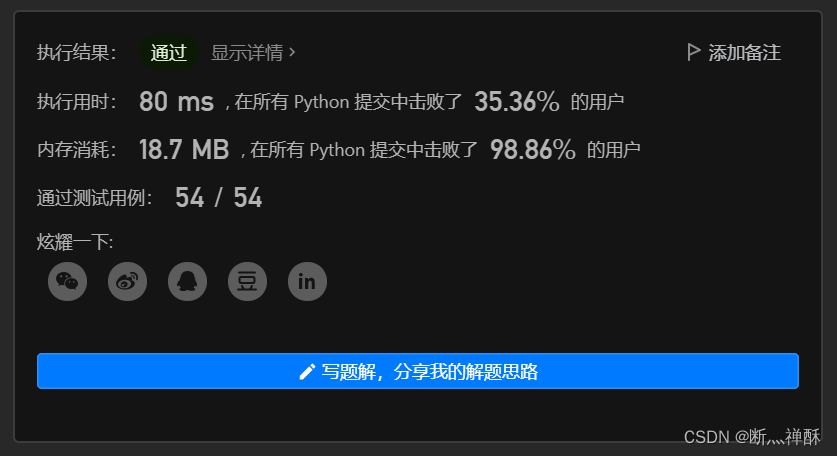# 代码对比

``````class Solution(object):
def containsNearbyDuplicate(self, nums, k):
queue = []
for i in nums:
if len(queue) > k:
queue.pop(0)    # 去头
if i in queue:
return True
queue.append(i)
return False````````````class Solution:
def containsNearbyDuplicate(self, nums, k):
window = set()
for i, num in enumerate(nums):
if len(window) == k + 1:
window.remove(nums[i - 1 - k])
if num in window:
return True
return False``````# 疑问

...全文
9 回复 打赏 收藏 举报python自主学习——Python基本数据结构（列表集合、元组）python列表, 元组, 集合以及字典的常见用法
python列表操作以及嵌套列表; 元组,集合,字典等常见操作python:列表、字典、元组与集合的特点以及差异
python:列表、字典、元组与集合的特点以及差异Day2 - Python基础2 列表、字典、集合
Python之路,Day2 - Python基础2 本节内容 列表、元组操作 字符串操作 字典操作 集合操作 文件操作 字符编码与转码 1. 列表、元组操作 列表是我们最以后最常用的数据类型之一，通过列表可以对数据实现最方便的存储、修改等操作 定义列表 1 names=['Alex',"Tengl...python列表、元组、集合手册
Content 列表操作 元组操作 集合操作 列表操作 列表python中一种可变可修改的数据类型，其可以存储所有的数据类型的信息。 ---- [ ] 中括号 列表的创建 　　列表有两种创建方式： 1 L = [1, 3, 4, 'Hello', True, print('3')] # 通过 [] 的方式创建列表 2 L =...

3.1w+

刷题！